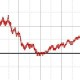# The Lumber Room

"Consign them to dust and damp by way of preserving them"

## The idea of logarithms, and the first appearance of e

The notion of the number$e$, the exponential function$e^x$, and logarithms$\log x$ are often conceptual stumbling blocks even to someone who has an otherwise solid understanding of middle-school mathematics.

Just what is the number$e$? How was it first calculated / where did it first turn up? Premature exposure to its numerical value$\displaystyle e \approx 2.718281828459045\dots$

only serves to deepen the mysteriousness and to make it seem arbitrary.

Here a historical perspective helps: as is often the case, here too, the first appearance is simpler and more well-motivated than the accounts in dry textbooks. This is from this account by Matthew P. Wiener (originally posted on USENET somewhere, as quoted by MJD). I’m just going to quote it directly for now, and edit it later:

Napier, who invented logarithms, more or less worked out a table of logarithms to base$\frac1e$, as follows:

     0  1  2  3   4   5   6    7    8    9    10 ...
1  2  4  8  16  32  64  128  256  512  1024 ...


The arithmetic progression in the first row is matched by a geometric progression in the second row. If, by any luck, you happen to wish to multiply 16 by 32, that just happen to be in the bottom row, you can look up their “logs” in the first row and add 4+5 to get 9 and then conclude 16·32=512.

For most practical purposes, this is useless. Napier realized that what one needs to multiply in general is$1+\epsilon$ for a base—the intermediate values will be much more extensive. For example, with base 1.01, we get:

       0 1.00   1 1.01   2 1.02   3 1.03   4 1.04   5 1.05
6 1.06   7 1.07   8 1.08   9 1.09  10 1.10  11 1.12
12 1.13  13 1.14  14 1.15  15 1.16  16 1.17  17 1.18
18 1.20  19 1.21  20 1.22  21 1.23  22 1.24  23 1.26
24 1.27  25 1.28  26 1.30  27 1.31  28 1.32  29 1.33
30 1.35  31 1.36  32 1.37  33 1.39  34 1.40  35 1.42
[...]
50 1.64  51 1.66  52 1.68  53 1.69  54 1.71  55 1.73
[...]
94 2.55  95 2.57  96 2.60  97 2.63  98 2.65  99 2.68
100 2.70 101 2.73 102 2.76 103 2.79 104 2.81 105 2.84
[...]


So if you need to multiply 1.27 by 1.33, say, just look up their logs, in this case, 24 and 29, add them, and get 53, so 1.27·1.33=1.69. For two/three digit arithmetic, the table only needs entries up to 9.99.

Note that$e$ is almost there, as the antilogarithm of 100. The natural logarithm of a number can be read off from the above table, as just [approximately]$\frac1{100}$ the corresponding exponent.

What Napier actually did was work with base .9999999. He spent 20 years computing powers of .9999999 by hand, producing a grand version of the above. That’s it. No deep understanding of anything, no calculus, and$e$ pops up anyway—in Napier’s case,$\frac1e$ was the 10 millionth entry. (To be pedantic, Napier did not actually use decimal points, that being a new fangled notion at the time.)

Later, in his historic meeting with Briggs, two changes were made. A switch to a base$> 1$ was made, so that logarithms would scale in the same direction as the numbers, and the spacing on the logarithm sides was chosen so that$\log(10)=1$. These two changes were, in effect, just division by$-\log_e(10)$.

In other words,$e$ made its first appearance rather implicitly.

(I had earlier read a book on Napier and come to the same information though a lot less clearly, here.)

I had started writing a series of posts leading up to an understanding of the exponential function$e^x$ (here, here, here), but it seems to have got abandoned. Consider this one a contribution to that series.

Written by S

Wed, 2013-11-27 at 10:52:51

Posted in mathematics

### 2 Responses

1. Looking forward to reading more about this. I never expected that e’s first appearance was as the limit of (1 + 1/n) ^ n – the order we learn these things really doesn’t match the history.loseyourmarblesblog

Thu, 2013-11-28 at 07:11:35

2. Very interesting historical background to e, thanks for sharing! Somehow the name Napier just keeps on popping up, but I can’t think of a single formula named after him. A shame really, he made great contributions to mathematics.allmediamath

Fri, 2013-11-29 at 00:30:11

This site uses Akismet to reduce spam. Learn how your comment data is processed.